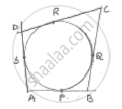Share

# In Fig. a Circle Touches All the Four Sides of Quadrilateral Abcd with Ab = 6cm, Bc = 7cm, Cd = 4cm. Find Ad. - CBSE Class 10 - Mathematics

ConceptCircles Examples and Solutions

#### Question

In fig. a circle touches all the four sides of quadrilateral ABCD with AB = 6cm, BC = 7cm, CD = 4cm. Find AD.

#### Solution

We know that the tangents drawn from any external point to circle are equal in length.From A ⟶ AS = AP ….(i)

From B ⟶ QB = BP …. (ii)

From C ⟶ QC = RC …..(iii)

From D ⟶ DS = DR …. (iv)

Adding (i), (ii), (iii) & (iv)

(AS + QB + QC + DS) = (AB + BP + RC + OR)

(AS + DS) + (QB + QC) = (AP + BP) + (RC + DR)

AD + BC = AB + CD